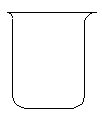# The Old Nassau (Gold and Black) Reaction

$$\newcommand{\vecs}{\overset { \rightharpoonup} {\mathbf{#1}} }$$ $$\newcommand{\vecd}{\overset{-\!-\!\rightharpoonup}{\vphantom{a}\smash {#1}}}$$$$\newcommand{\id}{\mathrm{id}}$$ $$\newcommand{\Span}{\mathrm{span}}$$ $$\newcommand{\kernel}{\mathrm{null}\,}$$ $$\newcommand{\range}{\mathrm{range}\,}$$ $$\newcommand{\RealPart}{\mathrm{Re}}$$ $$\newcommand{\ImaginaryPart}{\mathrm{Im}}$$ $$\newcommand{\Argument}{\mathrm{Arg}}$$ $$\newcommand{\norm}{\| #1 \|}$$ $$\newcommand{\inner}{\langle #1, #2 \rangle}$$ $$\newcommand{\Span}{\mathrm{span}}$$ $$\newcommand{\id}{\mathrm{id}}$$ $$\newcommand{\Span}{\mathrm{span}}$$ $$\newcommand{\kernel}{\mathrm{null}\,}$$ $$\newcommand{\range}{\mathrm{range}\,}$$ $$\newcommand{\RealPart}{\mathrm{Re}}$$ $$\newcommand{\ImaginaryPart}{\mathrm{Im}}$$ $$\newcommand{\Argument}{\mathrm{Arg}}$$ $$\newcommand{\norm}{\| #1 \|}$$ $$\newcommand{\inner}{\langle #1, #2 \rangle}$$ $$\newcommand{\Span}{\mathrm{span}}$$$$\newcommand{\AA}{\unicode[.8,0]{x212B}}$$

## Chemical Concept Demonstrated

• Chemical kinetics

## Demonstration

 Three solutions are combined together in alphabetical order.: Solution A: starch solution Solution B: HgCl2 solution Solution C: KIO3 solutionVideo 1: The attractiveness of Landolt reaction is mainly due to spectacular, even more unusual than in other reactions clock, the time course of processes, complicated its course, and the myriad possibilities in modifying the show.

## Observations

The resulting solution turns yellow, then turns black.

## Explanation (including important chemical equations)

This demonstration is a variant of the Landolt or iodine clock reaction.

$IO^-_{3(aq)} + 3 HSO^-_{3(aq)} \rightarrow I^-_{(aq)} + 3 SO^{2-}_{4(aq)} + 3 H^+_{(aq)} \tag{1}$

$IO^-_{3(aq)} + 5 I^-_{(aq)} + 6 H^+_{(aq)} \rightarrow 3 I_{2 (aq)} + 3 H_2O_{(l)} \tag{2}$

$I_{2 (aq)} + HSO^-_{3(aq)} + H_2O_{(l)} \rightarrow 2 I^-_{(aq)} + SO^{2-}_{4(aq)} + 3 H^+_{(aq)} \tag{3}$

$I_{2 (aq)} + I^-_{(aq)} + \text{starch}_{(aq)} \rightarrow \text{starch}-I^{3-}_{(aq)} \tag{4}$

The presence of HgCl2 means that a fifth (and perhaps sixth) reaction can occur.

$Hg^{2+}_{(aq)} + 2 I^-_{(aq)} \rightarrow HgI_{2 (s)} \tag{5}$

$HgI_{2 (s)} + 2 I^-_{(aq)} \rightarrow HgI^{2-}_{4(aq)} \tag{6}$

The yellow HgI2 precipitate forms as soon as the I - concentration is large enough for the ion product to exceed the solubility product for HgI2. Using the quantities specified above, the HgI2 precipitate does not dissolve. Instead, the yellow suspension of the HgI2 precipitate is masked by the appearance of the deep-blue-black starch-I3- complex when the HSO3- is exhausted.

An alternative form of the demonstration can be prepared by doubling the concentration of HSO3- in solution A. There is now enough HSO3- present initially to consume all of the IO3- ion. The HSO3- is never exhausted and the I -concentration can become high enough to redissolve the HgI2 precipitate. The solution, therefore, turns from clear to yellow and then back to clear again.

## Contributors

The Old Nassau (Gold and Black) Reaction is shared under a not declared license and was authored, remixed, and/or curated by LibreTexts.